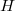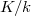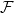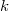﻿

### Minimal Hopf-Galois Structures on Separable Field Extensions

#### Abstract

In Hopf-Galois theory, every$H$-Hopf-Galois structure on a field extension$K/k$ gives rise to an injective map$\mathcal{F}$ from the set of$k$-sub-Hopf algebras of$H$ into the intermediate fields of$K/k$. Recent papers on the failure of the surjectivity of$\mathcal{F}$ reveal that there exist many Hopf-Galois structures for which there are many more subfields than sub-Hopf algebras. In this paper we survey and illustrate group-theoretical methods to determine$H$-Hopf-Galois structures on finite separable extensions in the extreme situation when$H$ has only two sub-Hopf algebras. This corresponds to the case when the lack of surjectivity is at its extreme.

DOI Code: 10.1285/i15900932v41n1p55

Keywords: Galois and Hopf-Galois field extensions; Galois correspondence; characteristically simple groups

Full Text: PDF